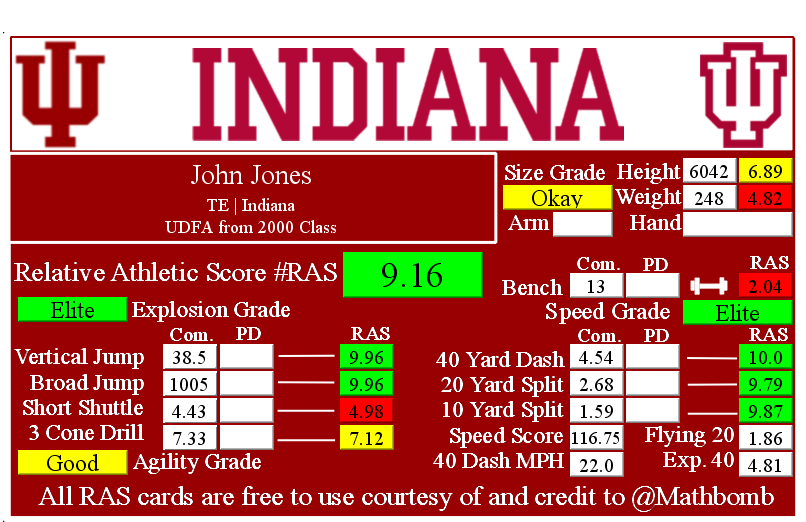# John Jones RAS

### John Jones RASJohn Jones went undrafted for the NFL in 2000.

He recorded a Relative Athletic Score of 9.16, out of a possible 10.0. RAS is a composite metric on a 0 to 10 scale based on the average of all of the percentile for each of the metrics the player completed either at the Combine or pro day.

He had a recorded height of 6042 that season, recorded as XYYZ where X is feet, YY is inches, and Z is eighths of an inch. That correlates to 6 feet, 4 and 2/8 of an inch or 76.25 inches, or 193.675 centimeters. This correlates to a 6.89 score out of 10.0.

He recorded a weight of 248 in pounds, which is approximately 112 kilograms. This correlates to a 4.82 score out of 10.0.

Based on his weight, he has a projected 40 yard dash time of 4.81. This is calculated by taking 0.00554 multiplied by his weight and then adding 3.433.
At the Combine, he recorded a 40 yard dash of 4.54 seconds. This was a difference of -0.27 seconds from his projected time. This forty time correlates to a 10.0 score out of 10.0.

Using Bill Barnwell’s calculation, this Combine 40 time gave him a Speed Score of 116.75.

The time traveled between the 20 and 40 yard lines is known as the Flying Twenty. As the distance is also known, we can calculate the player’s speed over that distance. The time he traveled the last twenty yards at the Combine was 1.86 seconds. Over 20 yards, we can calculate his speed in yards per second to 10.75. Taking into account the distance in feet (60 feet), we can calculate his speed in feet per second to 32.26. Breaking it down further, we can calculate his speed in inches per second to 387.1. Knowing the feet per second of 32.26, we can calculate the approximate miles per hour by multiplying that value by 0.681818 to give us a calculated MPH of 22.0 in the last 20 yards of his run.

At the Combine, he recorded a 20 yard split of 2.68 seconds. This correlates to a 9.79 score out of 10.0.

We can calculate the speed traveled over the second ten yards of the 40 yard dash easily, as the distance and time are both known. The time he traveled the second ten yards at the Combine was 1.09 seconds. Over 10 yards, we can calculate his speed in yards per second to 9.17. Taking into account the distance in feet (30 feet), we can calculate his speed in feet per second to 27.52. Breaking it down further, we can calculate his speed in inches per second to 330.28. Knowing the feet per second of 27.52, we can calculate the approximate miles per hour by multiplying that value by 0.681818 to give us a calculated MPH of 18.8 in the second ten yards of his run.

At the Combine, he recorded a 10 yard split of 1.59 seconds. This correlates to a 9.87 score out of 10.0 that when multiplied by ten roughly corresponds to percentile.

The time he traveled the first ten yards at the Combine was 1.59 seconds. Over 10 yards, we can calculate his speed in yards per second to 6.0. Taking into account the distance in feet (30 feet), we can calculate his speed in feet per second to 19.0. Breaking it down further, we can calculate his speed in inches per second to 226.0. Knowing the feet per second of 19.0, we can calculate the approximate miles per hour by multiplying that value by 0.681818 to give us a calculated MPH of 13.0 in the first ten yards of his run.

At the Combine, he recorded a bench press of 13 repetitions of 225 pounds. This correlates to a 2.04 score out of 10.0.

At the Combine, he recorded a vertical jump of 38.5 inches. This correlates to a 9.96 score out of 10.0.

At the Combine, he recorded a broad jump of 1005, which is recorded as FII or FFII . where F is feet and I is inches. This correlates to a 9.96 score out of 10.0 that when multiplied by ten roughly corresponds to percentile.

At the Combine, he recorded a 5-10-5 or 20 yard short shuttle of 4.43 seconds. This correlates to a 4.98 score out of 10.0.

At the Combine, he recorded a 3 cone L drill of 7.33 seconds. This correlates to a 7.12 score out of 10.0 that when multiplied by ten roughly corresponds to percentile.

This player did not record any measurements at their pro day that we were able to find. If they recorded Combine measurements, they stood on them.

This site uses Akismet to reduce spam. Learn how your comment data is processed.